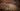# ECMAScript 提案：Array, 类数组和字符串的 .item() 方法

2020 年 11 月更新：名为 `.item()` 的方法已经结束支持。实验性的新名称是 `.at()`

## 可索引类的方法 `item()`

``````const arr = ['a', 'b', 'c', 'd'];
assert.equal(arr.item(1), 'b');
assert.equal(arr.item(0), 'a');
assert.equal(arr.item(-1), 'd');``````

``````arr.item(-1);
arr[arr.length - 1];``````

``````['a', 'b', 'c', 'd'].slice(1, -1);
// -> [ 'b', 'c' ]
['a', 'b', 'c', 'd'].slice(-1);
// -> [ 'd' ]``````

### 索引溢出

``````// Set up an Array
const arr = [];
arr['4294967296'] = 'abc';

// `arr` has no indexed properties
assert.equal(arr.length, 0);

// Index 4294967296 is out of bounds
assert.equal(arr, 'abc');``````

``assert.equal(arr.item(4294967296), undefined);``

### 拥有方法 `.item()` 的类

• Array
• 所有的类数组：`Uint8Array`
• string

• HTMLCollection（动态的，由 `.getElementsByClassName()``.getElementsByTagName()` 等返回）。
• NodeList（静态的，由 `.querySelectorAll()` 返回）。
• DOMTokenList（静态的，`.classList` 的值）。
• 其它情况

## 访问数组末端元素 - 可用于替换 `.item()`

``````const arr = ['a', 'b', 'c', 'd'];
const N = -2;

const element1 = arr[arr.length + N];
assert.equal(element1, 'c');

const element2 = arr.slice(N);
assert.equal(element2, 'c');

const { length, [length + N]: element3 } = arr;
assert.equal(element3, 'c');``````

``````const lastElement = arr.pop();
assert.equal(lastElement, 'd');``````

## `.item()` polyfill

``````function item(n) {
// ToInteger() abstract operation
n = Math.trunc(n) || 0;

// Allow negative indexing from the end
if (n < 0) n += this.length;

// Out-of-bounds access is guaranteed to return undefined
if (n < 0 || n >= this.length) return undefined;

// Otherwise, this is just normal property access
return this[n];
}

// Other TypedArray constructors omitted for brevity.
for (const C of [Array, String, Uint8Array]) {
Object.defineProperty(C.prototype, 'item', {
value: item,
writable: true,
enumerable: false,
configurable: true,
});
}``````

## `.item()` 和升级中的可索引 DOM 集合

``````// Old:
[...document.querySelectorAll('img')].map((img) => img.src);

// New:
document.querySelectorAll('img').map((img) => img.src);``````

## 例子：与 `.replace()` 回调函数一起使用

``````const result = 'first=jane, last=doe'.replace(
/(?<key>[a-z]+)=(?<value>[a-z]+)/g,
(...args) => {
const groups = args.item(-1); // (A)    const { key, value } = groups;
return key.toUpperCase() + '=' + value.toUpperCase();
},
);
assert.equal(result, 'FIRST=JANE, LAST=DOE');``````

`groups` 一直是 `.replace()` 回调函数的最后一个参数。如果在行 A 中 `.item()` 不可用，同样有效的是：

``const groups = args.pop();``

## 问题解释 FAQ

### 为什么不允许在括号中使用负值索引？

``````const english = ['hello', 'world'];
const german = ['hallo', 'Welt'];

function translate(word) {
return german[english.indexOf(word)];
}

assert.equal(translate('world'), 'Welt');
assert.equal(translate('universe'), undefined);``````

``````const arr = ['fee', 'fi', 'fo', 'fum'];
arr['-1'] = 'Englishman';

// Current behavior:
assert.equal(arr[-1], 'Englishman');``````

``````const numbers = [1, 2, 3];
const reversed = [];

let i = numbers.length - 1;
while (numbers[i]) {
reversed.push(numbers[i--]);
}

assert.deepEqual(reversed, [3, 2, 1]);``````